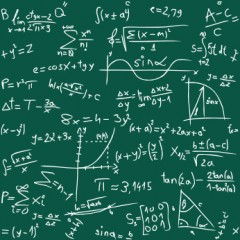# How Do You Do 4x + 3 = 2x + 11?To solve the equation 4x + 3 = 2x + 11 first the like terms need to be collected so we can isolate the x value and thus find x. To do this, arrange the x terms on one side of the equation and the integers on the other. When performing this action, remember that when a term crosses the equals sign it changes from positive to negative and vice versa.

Find x by collecting like terms.

4x + 3 = 2x + 11

Shift the 2x across the equals sign to collect like terms, it become -2x. Likewise with the 3, thus it becomes - 3. Now we have all the x terms on one side and the integers on the other. This leaves us with...

4x - 2x = 11 - 3

Then simplify by subtracting the 2x from the 4x to leave 2x, and subtract the 3 from 11 to get 8. Therefore...

2x = 8

Now we can divide both terms by 2 to get a value for x.

x = 4

To check the answer substitute the x value back into the equation, which leaves us with...

4(4) + 3 = 2(4) + 11

16 + 3 = 8 + 11

19 = 19   therefore 4 is the correct value for x.

thanked the writer.
The solution to the equation above is: 4x + 3 = 2x + 11; 4x - 2x = 11 - 3   2x = 8; x = 4.
thanked the writer.

1. I have no idea how to do this sooo....

1. Take the equation. 4x +3 = 2x +11.

2. Know what the question is asking you. (Im just taking a random guess) 4x is 16. 2x is 4!

3. The question is now 16 + 3 = 4 + 11.

4. 16 + 3 is 19 and 4 +11 is 15.

5. Add 19 and 15.... (34)

6. The answer is 34. (maybe idk)

So that concludes 4x +3 = 2x + 11= 34

thanked the writer.